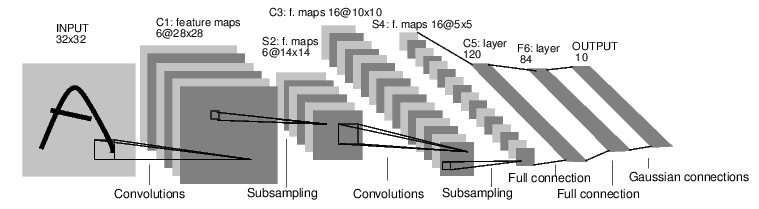# Neural Networks¶

Neural networks can be constructed using the torch.nn package.

Now that you had a glimpse of autograd, nn depends on autograd to define models and differentiate them. An nn.Module contains layers, and a method forward(input)that returns the output.

For example, look at this network that classfies digit images:convnet

It is a simple feed-forward network. It takes the input, feeds it through several layers one after the other, and then finally gives the output.

A typical training procedure for a neural network is as follows:

• Define the neural network that has some learnable parameters (or weights)
• Iterate over a dataset of inputs
• Process input through the network
• Compute the loss (how far is the output from being correct)
• Propagate gradients back into the network’s parameters
• Update the weights of the network, typically using a simple update rule: weight = weight - learning_rate * gradient

## Define the network¶

Let’s define this network:

import torch
import torch.nn as nn
import torch.nn.functional as F

class Net(nn.Module):

def __init__(self):
super(Net, self).__init__()
# 1 input image channel, 6 output channels, 5x5 square convolution
# kernel
self.conv1 = nn.Conv2d(1, 6, 5)
self.conv2 = nn.Conv2d(6, 16, 5)
# an affine operation: y = Wx + b
self.fc1 = nn.Linear(16 * 5 * 5, 120)
self.fc2 = nn.Linear(120, 84)
self.fc3 = nn.Linear(84, 10)

def forward(self, x):
# Max pooling over a (2, 2) window
x = F.max_pool2d(F.relu(self.conv1(x)), (2, 2))
# If the size is a square you can only specify a single number
x = F.max_pool2d(F.relu(self.conv2(x)), 2)
x = x.view(-1, self.num_flat_features(x))
x = F.relu(self.fc1(x))
x = F.relu(self.fc2(x))
x = self.fc3(x)
return x

def num_flat_features(self, x):
size = x.size()[1:]  # all dimensions except the batch dimension
num_features = 1
for s in size:
num_features *= s
return num_features

net = Net()
print(net)


You just have to define the forward function, and the backward function (where gradients are computed) is automatically defined for you using autograd. You can use any of the Tensor operations in the forward function.

The learnable parameters of a model are returned by net.parameters()

params = list(net.parameters())
print(len(params))
print(params.size())  # conv1's .weight


The input to the forward is an autograd.Variable, and so is the output.

input = Variable(torch.randn(1, 1, 32, 32))
out = net(input)
print(out)


Zero the gradient buffers of all parameters and backprops with random gradients:

net.zero_grad()
out.backward(torch.randn(1, 10))


Note

torch.nn only supports mini-batches The entire torch.nn package only supports inputs that are a mini-batch of samples, and not a single sample.

For example, nn.Conv2d will take in a 4D Tensor of nSamples x nChannels x Height x Width.

If you have a single sample, just use input.unsqueeze(0) to add a fake batch dimension.

Before proceeding further, let’s recap all the classes you’ve seen so far.

Recap:
• torch.Tensor - A multi-dimensional array.
• autograd.Variable - Wraps a Tensor and records the history of operations applied to it. Has the same API as a Tensor, with some additions like backward(). Also holds the gradient w.r.t. the tensor.
• nn.Module - Neural network module. Convenient way of encapsulating parameters, with helpers for moving them to GPU, exporting, loading, etc.
• nn.Parameter - A kind of Variable, that is automatically registered as a parameter when assigned as an attribute to a Module.
• autograd.Function - Implements forward and backward definitions of an autograd operation. Every Variable operation, creates at least a single Function node, that connects to functions that created a Variable and encodes its history.
At this point, we covered:
• Defining a neural network
• Processing inputs and calling backward.
Still Left:
• Computing the loss
• Updating the weights of the network

## Loss Function¶

A loss function takes the (output, target) pair of inputs, and computes a value that estimates how far away the output is from the target.

There are several different loss functions under the nn package . A simple loss is: nn.MSELoss which computes the mean-squared error between the input and the target.

For example:

output = net(input)
target = Variable(torch.arange(1, 11))  # a dummy target, for example
criterion = nn.MSELoss()

loss = criterion(output, target)
print(loss)


Now, if you follow loss in the backward direction, using it’s .grad_fn attribute, you will see a graph of computations that looks like this:

input -> conv2d -> relu -> maxpool2d -> conv2d -> relu -> maxpool2d
-> view -> linear -> relu -> linear -> relu -> linear
-> MSELoss
-> loss


So, when we call loss.backward(), the whole graph is differentiated w.r.t. the loss, and all Variables in the graph will have their .grad Variable accumulated with the gradient.

For illustration, let us follow a few steps backward:

print(loss.grad_fn)  # MSELoss


## Backprop¶

To backpropogate the error all we have to do is to loss.backward(). You need to clear the existing gradients though, else gradients will be accumulated to existing gradients

Now we shall call loss.backward(), and have a look at conv1’s bias gradients before and after the backward.

net.zero_grad()     # zeroes the gradient buffers of all parameters

loss.backward()



Now, we have seen how to use loss functions.

The neural network package contains various modules and loss functions that form the building blocks of deep neural networks. A full list with documentation is here

The only thing left to learn is:

• updating the weights of the network

## Update the weights¶

The simplest update rule used in practice is the Stochastic Gradient Descent (SGD):

weight = weight - learning_rate * gradient

We can implement this using simple python code:

learning_rate = 0.01
for f in net.parameters():


However, as you use neural networks, you want to use various different update rules such as SGD, Nesterov-SGD, Adam, RMSProp, etc. To enable this, we built a small package: torch.optim that implements all these methods. Using it is very simple:

import torch.optim as optim